Lompat ke konten Lompat ke sidebar Lompat ke footer

# Wireless power transfer basic theory (CHAPTER II)

### 1. Literature review

Nikola Tesla, at the beginning of the 19th century was probably the first person to discover and introduce the concept of sending electrical energy wirelessly to the world through the research he did. However, this did not automatically make his findings enthusiastically accepted by scientists at the time. On the contrary, the concept that Tesla discovered at that time was considered as something that is harmful to living organisms, considering the side effects that could be caused by the emitted electromagnetic field could cause various kinds of diseases for the organisms that were subjected to it. This is what gradually makes Tesla's research results generally tend to be forgotten for decades.

It was only later in 2007, a group of researchers from MIT (Massachusetts Institute of Technology) America conducted research on Wireless Power Transfer using almost the same principle as Tesla's concept but has a fundamental difference that if what Tesla is researching is power transfer electricity using long-distance radiation techniques such as microwaves and radio waves, then it is different with MIT, namely using the near field concept (near field) with a close distance between the sender and the receiver. In the end, the researchers succeeded in transferring electrical power wirelessly over a distance of more than 2 meters and a power of 60 watts, where the efficiency reached 40% (Kurs, 2007). The results of this study later became the turning point for the development of the WPT system.

Two researchers (Sibakoti & Hambleton, 2011), demonstrate the results of research that has been done on WPT and claim to have succeeded in designing a system to transmit power wirelessly from the sending coil to the receiving coil which is capable of turning on a 40 Watt lamp with a distance of 18 cm between coil.

In addition to the studies above, a researcher from South Korea (Hwang, 2011) also published a journal in which there are basic principles of this WPT technology. The basic principle of this technology (wireless power transfer) according to Hwang is that two separate coils with the same resonant frequency can form a resonant system based on high-frequency magnetic coupling and power exchange in high efficiency, where the effect of this coupling on objects -The objects around tend to be weak because they work at different frequencies. Then in 2012, which was stated in his final project, UNRAM Electrical Engineering Student, had succeeded in transferring electrical power which was then used to turn on the LED and charge the Nokia N95 HP battery as a load with a charging power of 2.18 mW with a distance of 5 cm. Which to get maximum power transfer over a longer distance, can be done by modifying the coil dimensions, increasing the number of turns and the type of inductor material used and increasing the capacity of the capacitor value in the sending circuit (Kusuma, 2012).

Wireless power transfer is also known as WPT (Wireless Power Transfer). Wireless electric power transfer or wireless energy delivery is the process of sending energy from a power source to an electrical load without going through a cable intermediary. This wireless energy transfer has the potential to be used to power electronic equipment that requires relatively little power (Wikipedia.org, 2012).

### 2. Wireless power transfer

The transfer of electrical power using air media can be used to distribute energy where the energy source and load are located far apart. One of the advantages of this technology is that it can penetrate objects in its path (except bimetallic materials) so that certain places that are generally not possible to send electrical power via cables, can be reached with this technology. But in research (MIT, LIPI, etc.), WPT still transmits energy in small amounts and at a distance that is not too far away. And its application is also on tools that require relatively small energy.Figure 2.1 Similarities in Principles in the WPT System

As another example, the principle of induction in a transformer (Figure 2.1), can transmit electrical power from one coil without touching the other coil, even though the distance is still very close. In addition to transformers, the principle of electromagnetic radiation in radio waves can also transmit electrical energy wirelessly, but because of their low efficiency, these radio waves only play an important role in the telecommunications world in transmitting information and cannot be used to transmit large amounts of electrical power (replacing the cable). Scientists have also tried to concentrate electromagnetic waves like lasers (doesn't spread like electromagnetic waves in radio waves), but this is also not practical and can even damage and harm humankind. Finally found a way to be able to transmit electrical energy wirelessly, namely by using the principle of magnetic resonance, where energy is transferred at the same frequency to the sender and receiver, so that it will not affect objects around which have different frequencies.

In a transformer (Figure 2.1), electric current flows into the primary coil and induces the secondary coil, these two coils are not in contact, but are in very close proximity. The efficiency level of the transformer will be greatly reduced if these two coils are kept away. In addition to the transformer, the electric toothbrush (Figure 2.2) also uses the same induction principle as the transformer, the electric toothbrush will recharge the battery if it is placed in its place.

The efficiency level of an electromagnetic induction can be increased by using a resonator circuit. This method is also known as resonance induction, which is widely used in medical equipment. Using this principle, a device has been successfully built that can transmit electrical power wirelessly, over a distance that is much different from traditional induction.

Direct radiation, using an antenna that is directed directly from the source to the receiver without any obstacle to shoot energy using radio frequencies. In this way, the energy that can be received by the receiving circuit is increased, but this method also has a direct impact on the organism and can be harmful. For this reason, this method also cannot be used in the delivery of electrical energy with large power such as for industry, or for the consumption of daily electronic equipment. However, in reality, this matter is still being studied and explored to be able to shoot energy from outer space to earth according to the concept of "solar space power" (Asimov, 1941), (Figure 2.3), and for defense needs as a lethal weapon that can shoot energy. from the sky to the battlefield.Figure 2.3 Solar Space Power Concept

As explained above, the concept of sending electrical power used in this thesis is very different from radio waves or direct radiation, because in the process of sending electrical power it does not require conditions that require that there are no barriers between the transmitter circuit and the receiver circuit.

### 3. Principle of Electromagnetic Induction

Magnetism and electricity are two natural phenomena whose processes can be reversed. When H.C. Oersted (1777-1851) proved that around a current-carrying wire there is a magnetic field (meaning that electricity creates magnetism), scientists began to think about the relationship between electricity and magnetism. In 1821 Michael Faraday (1791-1867) proved that a changing magnetic field can cause an electric current (meaning a magnet creates electricity) through a very simple experiment (Figure 2.4). A magnet that is moved in and out of a coil can produce an electric current in the coil.

Galvanometer is a tool that can be used to determine whether there is an electric current flowing. When a magnet is moved in and out of the coil, the galvanometer needle deviates to the right and to the left. The movement of the galvanometer needle indicates that the magnet that is moved in and out of the coil creates an electric current. Electric current can occur if at the ends of the coil there is an emf (electromotive force). The emf that occurs at the ends of the coil is called induced emf. Electric current only arises when the magnet moves. If the magnet is stationary in the coil, there is no electric current at the end of the coil.

4. The process of the occurrence of induced emf

When the north pole of the bar magnet is moved into the coil, the number of magnetic lines of force in the coil increases. This increasing number of lines of force causes an induced emf at the ends of the coil. The induced emf causes an electric current to flow moving the galvanometer needle. The direction of the induced current can be determined by observing the direction of the magnetic field it generates. When the magnet enters, the lines of force in the coil increase. As a result, the magnetic field produced by the induced current reduces the line of force. Thus, the end of the coil is the north pole so that the direction of the induced current is as shown in Figure 2.3(a).

When the north pole of the bar magnet is moved out of the coil, the number of magnetic lines of force present in the coil decreases. This reduction in the number of lines of force also causes an induced emf at the ends of the coil. The induced emf causes an electric current to flow and move the galvanometer needle. The same is true when the bar magnet enters the coil. When the magnet exits the line of force in the coil decreases. As a result, the magnetic field produced by the induced current is adding to the line of force. Thus, the end of the coil is the south pole, so the direction of the induced current is as shown in Figure 2.4 (b).

When the north pole of the bar magnet is stationary in the coil, Figure 2.4 (c), the number of magnetic lines of force in the coil does not change (fixes). Because the number of lines of force is constant, there is no induced emf at the ends of the coil. As a result, there is no electric current and the galvanometer needle does not move. So, induced emf can occur at both ends of the coil if there is a change in the number of magnetic lines of force (magnetic flux) inside the coil. The emf that arises due to a change in the number of magnetic lines of force in the coil is called induced emf. The electric current generated by the induced emf is called the induced current. The occurrence of induced emf and induced current due to a change in the number of magnetic lines of force is called electromagnetic induction.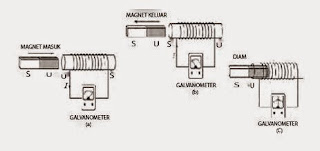Experiment 2.3 Faraday's Experiment

### 5. Factor of EMF

The size of the induced emf can be seen in the size of the deviation of the galvanometer needle angle. If the angle of deviation of the galvanometer needle is large, the induced emf and the resulting induced current are large. There are three factors that affect induced emf, namely:

1. The speed of movement of the magnet or the rate of change in the number of magnetic lines of force (magnetic flux)
2. Number of turns (N)
3. Magnetic field (B)

Like electric flux, magnetic flux can also be illustrated as the number of field lines that pass through a surface.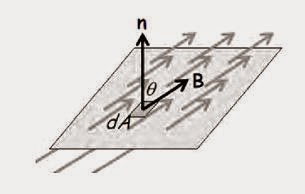Figure 2.4 Magnetic Flux

The electric flux produced by the field B on a surface of area dA is :

Experiments conducted by Faraday showed that changes in the magnetic flux on a surface bounded by a closed path will cause an emf. Faraday concludes that the magnitude of the emf that arises is:

### 6. Lenz .'s Law

The negative sign of Faraday's law is related to the direction of the induced emf. Lenz's law (1804-1865) states that the direction of the induced current is such that it creates an induced magnetic field that opposes the direction of the changing magnetic field.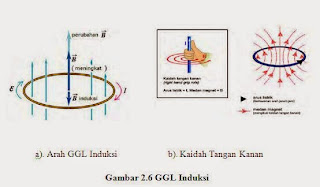GGL Induction

From Figure 2.6 (a) above, it can be seen that if the magnetic field increases (upwards), an induced magnetic field will arise in the opposite direction to the main magnetic field (downward), this induced field will produce an induced emf in the coil in the opposite direction. adjusted according to the right-hand rule as shown in Figure 2.6 (b).

### 7. SELF INDUCTION

From the explanation of the Biot-Savart law and Ampere's law (Jean Baptista Biot (1774–1862), Victor Savart (1803–1862), it has been shown that the presence of an electric current flowing in a conductor causes a magnetic field around the conductor. The magnet produced is proportional to the amount of electric current that flows, for example: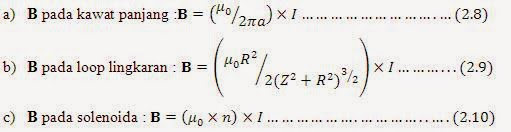Equations (2.8) to (2.10) show that B is proportional to I, and because from equation (2.2) it is obtained that B is proportional to theta , the magnetic flux is also proportional to the value of I. Therefore, the proportional constant can be obtained, namely :

Where L is the proportional constant between the flux and I which is called the (self) inductance of a system. Because in Faraday's law, changes in electric flux can cause emf, then equation (2.7) can be expressed by:

### 8. Joint Inductance

The current in coil 1 (Figure 2.9), will produce a magnetic field whose magnetic flux will affect coil 2. If it changes, then the magnetic field in coil 1 will also change, and this will cause an induced emf to occur in coil 2. in coil 2, the current will flow in coil 2 and will produce a magnetic field which will also affect coil 1, this is called the mutual inductance (M), which according to Faraday's law is: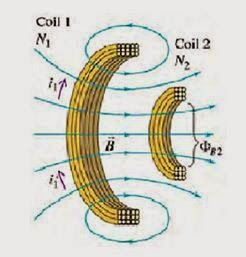Figure 2.9 Joint Inductance

### 9. Principle of Electromagnetic Resonance

The phenomenon of resonance is widespread in this realm. Different types of resonance also contain different energies. The sound of a tuning fork results from a resonance, as well as an earthquake results from a resonance, but the energy of an earthquake is much greater than the sound of a tuning fork.Figure 2.10 Tuning Fork Resonating

Resonance is a symptom of a system which in a frequency tends to absorb more energy from the environment. In other words, resonance is a phenomenon where if an object or object vibrates, then other objects with the same frequency will also vibrate. Resonance can transmit energy. As a simple example, if there are 2 tuning forks with the same frequency and sufficient distance, then if we hit the tuning fork A so that it makes a sound, then when we hold the tuning fork A until the sound stops, the tuning fork B will sound too even though it doesn't. we hit. This is an acoustic resonance phenomenon. The energy that makes the tuning fork B vibrate is generated from sound waves from the tuning fork A, the medium of which is the sound field. It can be said that the essence of this vibration propagation is a transmission of energy. Similar to sound fields, this can also be possible in electromagnetic fields.

### 10. Electromagnetic Resonance

Electromagnetic resonance exists widely in electromagnetic systems. The electromagnetic field itself is an energy field that can provide energy to be used in the process of electricity flow. Considering the danger to people and other organisms in electric fields, magnetic fields are safe and more suitable to be used as energy delivery media in magnetic resonance energy transfer.

Electromagnetic waves are waves that can propagate even though there is no medium and consist of an electric field and a magnetic field as illustrated in Figure 2.11. Electromanetic wave radiation itself contains energy. No matter whether there is a receiver or not, the energy of the electromagnetic wave is continuously consumed. If we can create a non-radiating magnetic field with a certain resonant frequency, the inductance coil will continue to collect energy, the receiver voltage will increase, and the received energy can be transmitted to the load after being converted by an additional circuit.Figure 2.11 Direction of Electromagnetic Wave Propagation

In general, electromagnetic systems with the same resonant frequency have weaknesses within a certain range. Two systems with the same resonant frequency will produce a strong magnetic resonance and form a magnetic resonance system. If there are more than two resonant generators in the effective range, they can also join this magnetic resonance system. One resonator can be connected to a continuous power supply to act as an energy source and the other consumes energy, so that this energy delivery system can be realized. In other words, this system can transmit energy from one place to another via an invisible magnetic field (wireless), rather than in the usual way through visible electrical wires.

### 11. Mutual Resonance Principle

The basic principle of electromagnetic induction is when an alternating current passes through a coil, around the coil will produce a magnetic field. If in this condition another coil is placed near the coil, the magnetic field from the first coil will also arise around the second coil. This is the reason why wireless energy transfer can occur between the two coils. As previously described, mutual resonance is a special state of wireless energy delivery. The location of the specialty is that all the coils used to resonate together operate under resonant conditions.Figure 2.12 Joint Resonance

Resonance occurs when the self-resonant frequency of the coils is equal to the frequency of the alternating current source, when the equivalent circuit of the coils at high frequencies has the smallest impedance. At times like this, the most energy can be sent through the resonance path. Figure 2.12 shows the occurrence of a joint magnetic resonance process, the yellow color indicates a coil that has the same resonant frequency, the blue and red colors indicate the magnetic field caused in the coil, both of which are identical to each other, this is a simple description of the mutual resonance.

### 12. LC circuit

The LC circuit (Figure 2.13) is a resonant circuit consisting of an inductor (L) and a capacitor (C). LC circuits are commonly used to generate alternating current sources or as signal generators.Figure 2.13 LC . circuit

#### 12.5.1 LC Circuit Working Principle

The working principle of the LC circuit in order to produce alternating or oscillating signals is to use capacitors and inductors. Capacitors store energy in the electric field between the two plates, based on the magnitude of the voltage between the two plates, while inductors store energy in the magnetic field, based on the magnitude of the current through the inductor. Figure 2.14 describes the working principle of the LC circuit.Figure 2.14 Working Principle of LC . Circuit

In Figure 2.14 above, the leftmost position shows the beginning, t = 0 or t = T, where the capacitor value is maximum, and no current flows. When the switch starts to close, which is between t = 0 to t = T/4, a closed circuit occurs, the capacitor begins to discharge, and the current flows counterclockwise to the inductor and continues to increase.

At the condition t = T/4, the capacitor has a minimum value, the maximum current flows and is still counterclockwise. From t = T/4 to t = T/2, current continues to flow charging the capacitor with the opposite side, and the current flowing begins to decrease.

At time t = T/2, no more current flows in the circuit, and the maximum capacitor. From t = T/2 to t = 3T/4, the capacitor begins to discharge, and the current flows clockwise and continues to increase.

At time t = 3T/4, the capacitor is empty, the maximum current flows through the inductor clockwise.

From t = 3T/4 to t = T, the capacitor starts to charge again, current goes to the capacitor with the same side as the starting side in a clockwise direction and continues to decrease until the capacitor is full.

This continues to repeat to the beginning, so that the signal is obtained back and forth.

12.5.2 LC Rangkaian Circuit Oscillation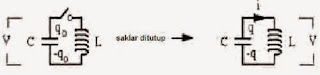Figure 2.15 Oscillation of LC . Circuit

Referring to Figure 2.15, when the switch is closed, the voltage across the capacitor and inductor is the same.

Adjust both sides, and we know that i = -dq/dt, so that equation 2.19 can be written,

If we give a constraint where i = 0, when t = 0, because the current (inductor cannot change directly), then we can determine the value of the capacitance of the capacitor when it is charging by integrating the current (i) and multiplying -1, then we get ,

### 12.6 Cordless Power Delivery StructureFigure 2.17 Schematic of Electrical Power Delivery Diagram without cables

In Figure 2.17 above, a simple schematic diagram of a wireless power delivery system is shown using the principle of magnetic resonance induction. The block on the left (marked with a dotted line) is a transmitter circuit, while the block on the right is a receiver circuit for the system. At the transmitter, the alternating current power source is rectified first with a DC module, then enters the LC circuit, in this case Ls and Cs, to create a non-radiative alternating magnetic field signal generator. The resonant frequency of this LC circuit is called.

On the side of the receiver circuit, there is also an LC circuit, where Lt and Ct function to generate resonance from the magnetic field generated by the transmitter circuit to receive electrical power. The frequency of the receiving circuit is called.

The value of the magnetic resonance frequency at the receiving end is largely determined by the magnetic resonance frequency in the transmitter circuit. The closer the value is to or equal to, the greater the value of the resonant current, the stronger the magnetic field and the greater the electrical power that can be received or transmitted.

In the receiver circuit, it should be emphasized that the LC circuit does not have to be the same to transmit electrical power, as long as the resonant frequency is the same ( = ), then a wireless electric power delivery system using magnetic resonance induction can still work.

The farther the distance from the transmitter circuit to the receiver circuit, the smaller the electrical power that can be received by the receiver circuit.

The process of sending electrical power without wires can still transmit electrical power even though it is blocked by various non-metallic objects, but if it is blocked by metal, then the electrical power cannot be received by the receiving circuit.

## 13 komentar untuk "Wireless power transfer basic theory (CHAPTER II)"

1.Makasih infonya bro.....
Saya suka elektronik dn kali ini lagi butuh info tentang alat atau rangkaian yg bs mengubah gelombang elektromagnetik jadi listrik. Simpelnya antena penangkapnya baiknya seperti apa desainya....thanks.

2.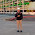dear mas sugeng, kalo ditanya mengenai design rceiver nya ya trgantung transmitter nya sih mas. Krn ini kan menggunakan prinsip resoonansi magnetik, di mana frequensi di sisi penerima sama dengan pengirim

3.Bagaimana menambah jauh aliran listrik tanpa kabel

1.Yang saya pahami caranya adalah memperbesar medan magnetnya

4.Bagaimana menambah jauh aliran listrik tanpa kabel

5.Apakah besar magnet yang bisa menjauhkan listrik atau lilitan logam yang bisa menambahkan listrik,,,

6.Dear mb Aryati, untk meanmbah jarak transfer listrik tanpa kabel, yg paling mendasar untk diperhatikan adalah deaign transmitter dan receiver nya

7.bang,untuk perancangan pengiriman energi listrik tanpa kabel kita harus mengetahui diameter kawat,diameter lingkaran pada kumparan dan berapa banyak lilitan yang kita butuhkan. bagaimana untuk mengatahuinya supaya perancangan yang kita buat itu bagus bang ? dan bagusnya brp diameter yang dibutuhkan dan jumlah lilitannya bang ?

8.contohnya untuk menghidupkan sebuah LED atau elektronik lainnya yang membutuhkan tegangan 12V dan 14watt

9.Boby@, coba baca bab 4 saya sob. Jawaban pertnyaanya sharusnya ada di situ. Inti dari sebuah lilitan yg akan kita buat (baik transmitter maupun receiver) adalah nilai induktansi lilitan dalam henry. Jika kita sudh menetapkan nilai induktansi yb kita perlukan, maka nyari parameter2 yg lain gampang

10.Boby@, coba baca bab 4 saya sob. Jawaban pertnyaanya sharusnya ada di situ. Inti dari sebuah lilitan yg akan kita buat (baik transmitter maupun receiver) adalah nilai induktansi lilitan dalam henry. Jika kita sudh menetapkan nilai induktansi yb kita perlukan, maka nyari parameter2 yg lain gampang

11.maaf bang,saya masih bingung. bisa mintak contact personnya bang,karna banyak hal yang ingin saya tanyakan. mohon bantuannya bang.

1.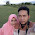Siapp,,